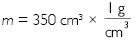# NCERT Solutions for Class 9 Science Chapter 10 - Gravitation

##### Question 1:

State the universal law of gravitation.

Newton’s universal law of gravitation states that every object in the universe attracts every other object with a force which is directly proportional to the product of their masses and inversely proportional to the square of the distance between them.
If m1 and m2 are the masses of two objects lying at a distance d apart, then force F between them is :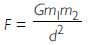where, G is a constant called universal gravitational constant.

##### Question 2:

Write the formula to find the magnitude of the gravitational force between the earth and an object on the surface of the earth.

Let m be the mass of an object on the earth’s surface and let mass of the earth be M. If R is the radius of the earth, the gravitational force between the earth and the object is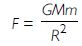where, G is a constant called universal gravitational constant and its value is 6.67 x 10-11 Nm2kg -2.

##### Question 3:

What do you mean by free fall?

An object is said to be in a state of free fall when it falls towards the earth only due to the gravitational force of the earth.

It falls towards the centre of the earth with an acceleration of 9.8 ms-2 (usually called acceleration due to gravity).

##### Question 4:

What do you mean by acceleration due to gravity?

Acceleration due to gravity is the acceleration with which a body falls due to gravitational force of the Earth.

It is represented by g and its value is 9.8 ms-2.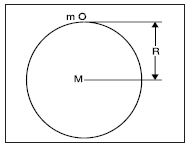If a mass m is placed on the earth with mass M and radius R, then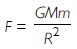But this force is also equal to the weight of the body i.e., F = mg

\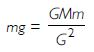or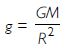##### Question 5:

What are the differences between the mass of an object and its weight?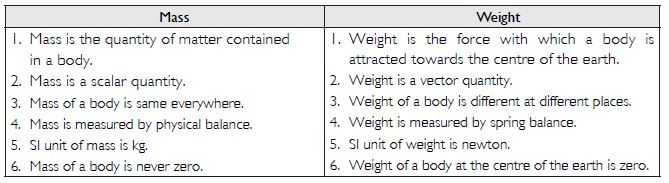##### Question 6:

Why is the weight of an object on the Moon 1/6th of its weight on the Earth?

Let m be the mass of an object. Let its weight on the moon be Wm.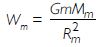where Mm and m are the mass and the radius of the moon respectively.
If mass and radius of the earth are Me and Re respectively, then the weight of the body We with mass m on the earth is: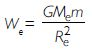From (i) and (ii), we have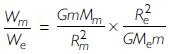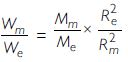Now Mm = 7.36 x 1022 kg ; Me = 5.98 x 1024 kg ;
Re = 6.37 x 106 m and Rm = 1.74 x 106 m

\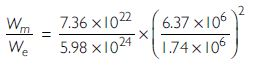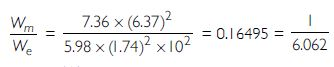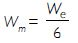Hence, weight of a body on the moon is 1/6th that on the earth.

##### Question 7:

Why is it difficult to hold a school bag having a strap made of thin and strong string?

As the pressure exerted by an object is force per unit area.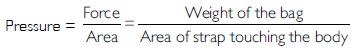Thus, a thin strap of small area will exert a large pressure on the student. Hence, it is difficult for the student to hold the bag with thin string.

##### Question 8:

What do you mean by buoyancy?

When a body is put in a liquid or gas, it experiences an upward force. Due to this upward force, substances appear lighter when immersed wholly or partially in a liquid. The tendency of a fluid (liquid or gas) to exert an upward force on the object is called buoyancy. The upward force that acts on the object is called buoyant force.

##### Question 9:

Why does an object float or sink when placed on the surface of water?

When a body is immersed in water, two forces are acting on it:
(i) Weight, W, of the body acting vertically downwards.
(ii) Upward thrust, w, equals to the weight of water displaced.
Three cases arise:
(i) If W > w, i.e., the downward force due to weight of the body is greater than weight of the water displaced, the body will sink. Iron, stone etc., sink in water because W > w.
(ii) If W > w, the body will remain in equilibrium anywhere inside water. In this case, the net weight of the body in the water is zero. This happens when the density of the body is exactly equal to density of water displaced.
(iii) If W > w i.e., the weight of the body is less than the weight of water displaced, the body will be forced upwards. As it projects above the surface of water, volume of water (and hence weight of water) displaced will become less. The upward force will go on decreasing till it becomes equal to weight of the body. Now, the body starts floating.

##### Question 10:

You find your mass to be 42 kg on a weighing machine. Is your mass more or less than 42 kg?

When we stand on a weighing machine, air exerts on upward force which makes us feel lighter than the real situation. Therefore, if in weighing machine, our mass is 42 kg, then our actual mass is more than 42 kg.

##### Question 11:

You have a bag of cotton and an iron bar indicating a mass of 100 kg when measured on a weighing machine. In reality, one is heavier than the other. Can you say which one is heavier and why?

Weighing machine measures weight and not the mass of the body. If the weight of cotton and iron is each equal to 100 kg, the real weight of cotton is more than that of iron. Due to larger volume of the cotton, the loss of weight when cotton is weighed in air is more than that of iron weighed in air. Therefore, the real weight (weight in vacuum) is more in case of cotton than that of iron.

##### Question 12:

How does the force of gravitation between two objects change when the distance between them is reduced to half?

Force of attraction between two objects is inversely proportional to square of the distance between the objects, therefore, force of attraction will become four times when distance between them is reduced to half.

##### Question 13:

Gravitational force acts on all objects in proportion to their masses. Explain why a heavy object does not fall faster than a light object.

Heavier bodies experience gravitational force in proportion to their masses but the acceleration with which they fall towards the earth is same for all bodies either heavier or lighter. Acceleration due to gravity g is same for all bodies.
We know that v = u + at = u + gt. As u = 0, v = gt. Since g is independent of the mass of the bodies, the velocity of all bodies will be equal after equal interval of time.

##### Question 14:

What is the magnitude of the gravitational force between the earth and a 1 kg object on its surface? Mass of the earth to be 6 x 1024 kg and radius of the earth is 6.4 x 106 m.

G = 6.67 x 10-11 Nkg-2m2; m1 = 1 kg ; m2 = 6 x 1024 kg ;
R = 6.4 x 106 m ; F = ?

F =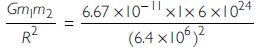F =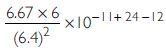F = 0.977 x 101 = 9.77 N

##### Question 15:

The earth and the moon are attracted to each other by gravitational force. Does the earth attract the moon with a force that is greater or smaller or the same as the force with which the moon attracts the earth? Why?

The earth and the moon attract each other with an equal force. The magnitude of force on each of the two bodies (moon and earth) is the same but the direction is opposite.

##### Question 16:

If the moon attracts the earth, why does the earth not move towards the moon?

Force of attraction due to gravitation provides the necessary centripetal force to the moon to enable it to move round the earth in almost a circular path. Acceleration is inversely proportional to mass of the Earth which is very large; therefore, acceleration produced in the earth is so small that it cannot be observed. Therefore, we cannot see the earth moving towards the moon.

##### Question 17:

What happens to the force between the two objects if:
(i) the mass of one object is doubled?
(ii) the distance between the objects is doubled and tripled?
(iii) the masses of both the objects are doubled?

The force of attraction between two objects of mass m1 and m2 lying at a distance d apart is given by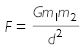(i) When mass of one of the objects (say m1 or m2) is doubled, F will be doubled.
(ii) When distance (d) between the two objects is doubled, F becomes one-fourth i.e., 1/4 times.
When distance (d) between the two objects is tripled (made 3 times), the force F between them is reduced to 1/9th of the original.
(iii) When both m1 and m2 are doubled, F becomes four times.

##### Question 18:

What is the importance of the universal law of gravitation?

The universal law of gravitation successfully explains that
(i) all bodies, small or large attract each other.
(ii) the gravitational force binds us to the earth.
(iii) the motion of the moon around the earth is due to gravitational force.
(iv) the motion of the earth and other planets around the sun is due to gravitational force.
(v) Artificial satellites move around the earth due to gravitational force.

##### Question 19:

What is the acceleration of free fall?

Acceleration of free fall is the acceleration with which a body falls towards the earth due to gravitational attraction of the earth. Its value is 9.8 ms-2.

##### Question 20:

What do we call the gravitational force between the earth and an object ?

It is called gravity.

##### Question 21:

Amit buys few grams of gold at the poles as per instruction from one of his friends. He hands over the same when he meets him at the equator. Will the friend agree with the weight of gold bought? If not, why?

(Hint: The value of g is greater at the poles than at the equator)
The value of g is more at the poles than at the equator. Due to the elliptical shape of the earth, the radius of the earth is more at the equator than at the poles.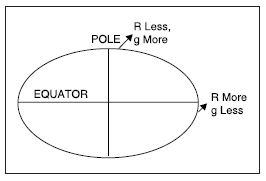Now,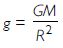Thus, more R, lesser g ; lesser R, greater g.
Weight = mg hence, gold will weigh lesser at the equator. The friend at the equator will not agree as he is getting lesser weight of gold.

##### Question 22:

Why will a sheet of paper fall slower than one that is crumpled into a ball?

A sheet of paper experiences larger force of friction due to air than a sheet that is crumpled into a ball. Area of contact between sheet and air is larger than the area of contact between air and crumpled sheet.
Increased force of friction will reduce the forward driving force more in case of sheet of paper than ball of crumpled sheet and hence, sheet will fall slower.

##### Question 23:

Gravitational force on the surface of the moon is 1/6th as strong as gravitational force on the earth. What is the weight in newtons of a 10 kg object on the moon and on the earth?

Mass m = 10 kg, ge (on the earth) = 9.8 ms-2
Weight on the earth = mge = 10 x 9.8 = 98 N
Since gravitational force on the moon is 1/6th of that on the earth, g on the moon is also 1/6th of that on the earth

gm (on the moon) =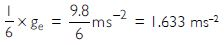Weight (on the moon) = mgm = 10 x 1.633
Wm = 16.33 N

##### Question 24:

A ball is thrown vertically upwards with a velocity of 49 ms-1, calculate
(i) the maximum height to which it rises.
(ii) the total time it takes to return to the surface of the earth..

u = 49 ms-1; a = - 9.8 ms-2 [going up];
v = 0 ms-1 [at top],
s = h = ? ; t = ?
Using v2 - u2 = 2as, we have
(0)2 - (49)2 = 2 (- 9.8)h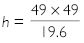h = 122.5 m
Using v = u + at, we have
0 = 49 - 9.8 t
or 9.8 t = 49

or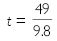t = 5 s which is the time of ascent (going up).
Since, time of ascent = time of descent
\ Total time = 2t = 2 x 5 = 10 s

##### Question 25:

A stone is released from the top of a tower of height 19.6 m. Calculate its final velocity just before touching the ground.

u = 0; s = 19.6 m; a = 9.8 ms-2; v = ?
Using v2 - u2 = 2as, we have
v2 - (0)2 = 2 (9.8) (19.6) = (19.6)2
v = 19.6 ms-1

##### Question 26:

A stone is thrown vertically upward with an initial velocity of 40 ms-1. Taking g = 10 ms-2, find the maximum height reached by the stone. What is the net displacement and total distance covered by the stone?

u = 40 m
a = - 10 ms-2; s = h = ?
v = 0 ms-1 [at maximum height]
Using v2 - u2 = 2 as, we have
(0)2 - (40)2 = 2 (-10) h
h = 80 m
Maximum height attained = 80 m
Stone goes 80 m upwards and then returns to the point of throw by moving 80 m downwards.
\ Total distance covered = 80 + 80 = 160 m
Since it returns to the point of throw, therefore net displacement is zero.

##### Question 27:

Calculate the force of gravitation between the earth and the sun, given the mass of the earth = 6 × 1024 kg and mass of the sun = 2 × 1030 kg. The average distance between the two is 1.5 × 1011m.

F = ?; m1 = 6 × 1024 kg; m2 = 2 × 1030 kg; d = 1.5 × 1011 m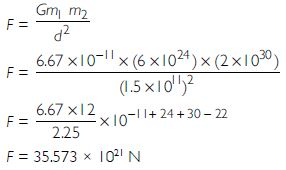##### Question 28:

A stone is allowed to fall from the top of a tower 100 m high and at the same time another stone is projected vertically upwards from the ground with a velocity of 25 ms-1. Calculate when and where the two stones will meet.

Let the stones meet at a distance ‘x’ metres from the top.
Considering downward motion;
u = 0; s = x, a = 10 ms-2

Using s =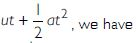x =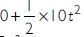x = 5 t2... (i)
Considering upward motion (of second stone), we have
u = 25 ms-1, a = - 10 ms-2, s = (100 - x) m

Using s =100 - x =But from (i) 5 t2 = x [from (i)]
\ 100 - x = 25t - x
or t = 4s
From (i), we have x = 5 (4)2 = 80 m
\ The stones will meet at a distance 80 m from the top.

##### Question 29:

A ball thrown up vertically returns to the thrower after 6 s. Find (i) velocity with which it was thrown up, (ii) the maximum height it reaches (iii) its position after 4 s.

(i) Since, time of ascent = time of descent
\ Time of ascent (going up to the top) =
Considering upward motion,
u = ?, a = - 9.8 ms-2, v = 0 ms-1 [at top]; t = 3 s , s = h = ?
Using v = u + at, we have
0 = u - 9.8 × 3 = u - 29.4
u = 29.4 ms-1

(ii) Using s =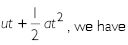h =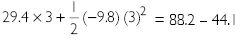h = 44.1 m
(iii) It took 3 s to reach the top. After 4 seconds from start, the ball was on downward journey for
4 - 3 = 1 s. Considering downward motion,
u = 0 ms-1; t = 1s; a = 9.8 ms-2; s = ?

s =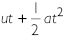=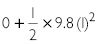s = 4.9 m below the top

##### Question 30:

In what direction does the buoyant force on an object immersed in a liquid act?

The buoyant force acts on an object immersed in a liquid in the vertically upward direction.

##### Question 31:

Why does a block of plastic released under water come up to the surface of water?

The weight of water displaced by a plastic block is more than its own weight. Upward force acting on the plastic block is therefore more than the downward force due to weight of the block. Due to excessive upward buoyant force, the block is forced upwards.

##### Question 32:

The volume of 50 g of substance is 20 cm3. If the density of water is 1 gcm-3, will the substance float or sink?

Density of substance =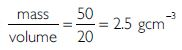The density of substance (2.5 gcm-3) is more than that of water (1 gcm-3). Therefore, the substance will sink to the bottom.

##### Question 33:

The volume of a 500 g sealed packet is 350 cm3. Will the packet float or sink in water if the density of water is 1 gcm-3? What will be the mass of water displaced by this packet?

Density of packet =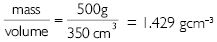i.e.,